Courses

# Chapter 3 - Feedback characteristics of control system - Notes, Control System, Electrical Engineeri Electrical Engineering (EE) Notes | EduRev

## Electrical Engineering (EE) : Chapter 3 - Feedback characteristics of control system - Notes, Control System, Electrical Engineeri Electrical Engineering (EE) Notes | EduRev

The document Chapter 3 - Feedback characteristics of control system - Notes, Control System, Electrical Engineeri Electrical Engineering (EE) Notes | EduRev is a part of the Electrical Engineering (EE) Course Electrical Engineering SSC JE (Technical).
All you need of Electrical Engineering (EE) at this link: Electrical Engineering (EE)

## FEEDBACK CHARACTERISTICS OF CONTROL SYSTEM

• We shall now investigate the effects of negative feedback on the various aspects of system performance. Following are listed the effect of negative feedback:

⇒ Effect of parameter variation reduces.
⇒ The gain of system reduces by a factor (1 + GH)
⇒ The bandwidth of system increases.
⇒ Effect of internal disturbances reduces.

SENSITIVITY (to parameter variation)
•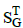: Is the ratio of percentage change in T to the percentage change in G.
where,

T = Transfer function
G = Forward path gain=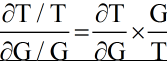(a) Open Loop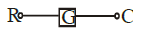One of the primary purpose of using feedback in control systems is to reduce the sensitivity of the system to parameter  ariations

T = C/R = G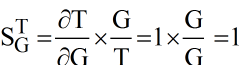= 1

Hence in open - loop system= 1(unity)

(b) Closed Loop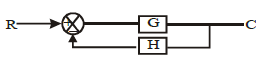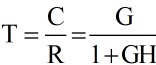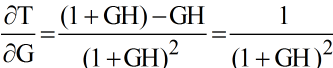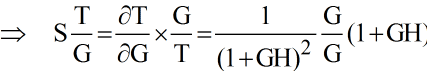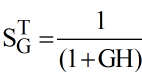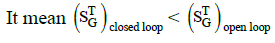Hence closed loop system is lesser sensitive to parameter variations; therefore closed loop system is better.

Sensitivity with respect to H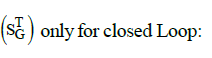• The term systems sensitivity is used to describe the relative variation in the overall transfer function T (s) = C(s) due to variation  in G(s) and is defined below: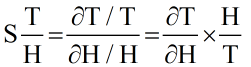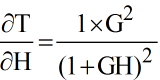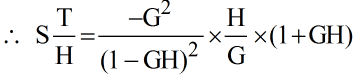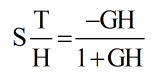Approximation: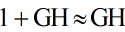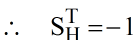Sensitivity with respect to H is high.

Note:
⇒ Feedback path contains measuring devices which are low power devices and these devices and these devices are more prone to external environmental effects and because of high sensitiveity towards feedback path gain the output of the system can change to undesirable level herefore feedback path must be provided with a shielding.
⇒ Forward path devices are high power devices and therefore they are lesser sensitive towards external changes so shielding so shielding is not important for forward path components or devices.
⇒ Feedback always introduces the possibility of instability in the system, meaning thereby that for an open loop system; closed system can be stable or unstable.

Effect of Disturbance (Noise) Signals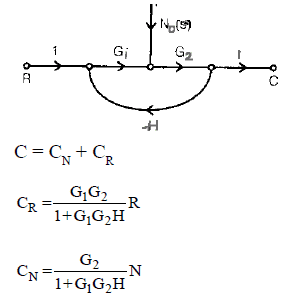Aim: To minimise CN

• Best way to do this is to increase G1. So the effect of noise can be reduced by increasing the forward path gain earlier to the noise.

REGENERATIVE FEEDBACK

• The precending material in this chapter has emphasized a negative or degenerative typed feedback. In regenerative feedback, the output is feedback with positive sign as shown in figure.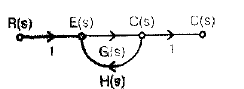• In this case, the transfer function is given by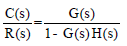• There is a negative sign in the denominator of equation (3.1) which indicates the possibility of denominator becoming equal to zero thereby giving ian infinite output for a finite input which is the condition of instability.
• The regenerative feedback is sometimes used for increasing the loop gain of feedback systems, figure shows a feedback system with an inner loop having regenerative feedback. This single flow graph reduces to a single loop graph whose loop gain is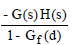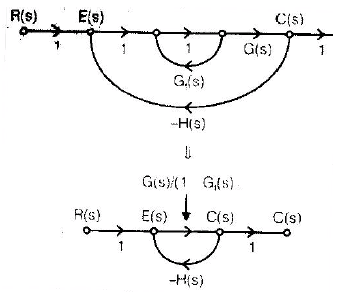• If Gf(s) is selected to be nearly unity, the loop gain becomes very high and the closed-loop transfer function approximates to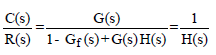• Thus due to high loop gain provided by the inner regenerative feedback loop, the closed-loop transfer function becomes insensitive to G(s).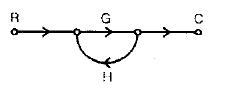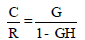Offer running on EduRev: Apply code STAYHOME200 to get INR 200 off on our premium plan EduRev Infinity!

65 docs|37 tests

,

,

,

,

,

,

,

,

,

,

,

,

,

,

,

,

,

,

,

,

,

,

,

,

,

,

,

;